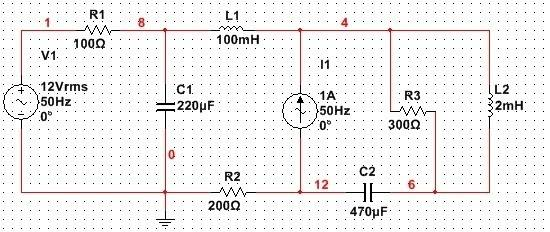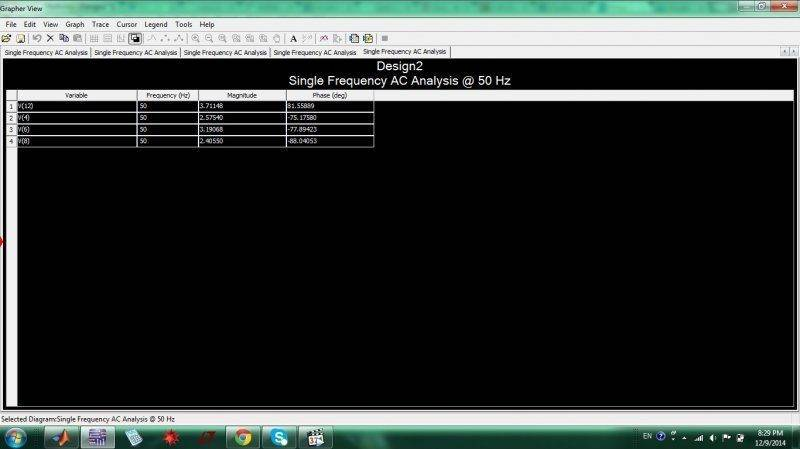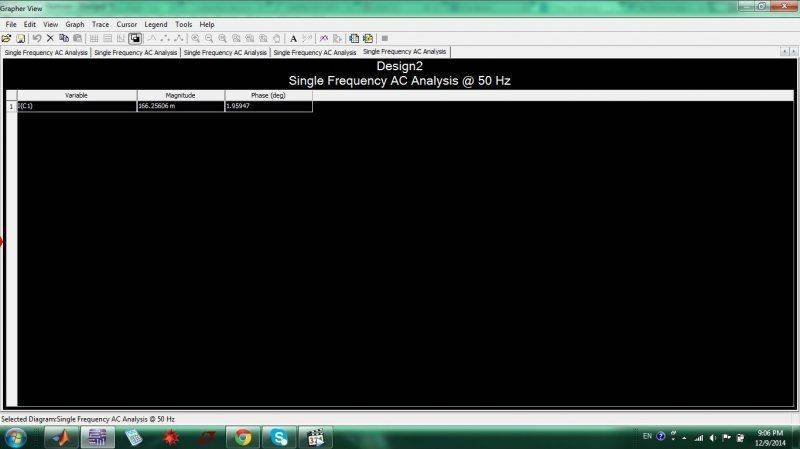# Phase measurement in Multisim

Hi!
Is it possible to measure phase angle in Multisim? I want to measure phase angle of current through capacitor C1:I got by hand ic1(t)=0.117*sqrt(2)*sin(2*pi*50*t-178.040deg)

Solved...

berkeman
Mentor
How did you do it?

Firstly, it's necessary to modify "AC analysis magnitude" of sources: in my case I put 16.970562 (12sqrt(2)) for voltage source, current source shouldn't be modified. Then I go to Simulate->Analysis->Single frequency AC analysis and there we choose Magnitude/Phase for Complex number format and in Output tab we select our variables. Here are phases for 4 nodes in our circuit:It matches with result I got by hand. However, for some reason phase of current through capacitor C1 doesn't match with my result.

It doesn't calculate phase angle on same way as I. Here is complex current IC1:
IC1=-0.1174-0.0040195j. Phase angle of this current is atan(-0.0040195/(-0.1174))-pi=-3.1073rad=-178.040deg. Multisim calculates only atan(-0.0040195/(-0.1174))=1.959deg.Last edited: